Contact

Aerospace & Defense

# Investigation of a Class-J Power Amplifier with a Nonlinear Cout for Optimized Operation

Junghwan Moon, Student Member, IEEE, Jungjoon Kim, and Bumman Kim, Fellow, IEEE
Nov 01, 2010
• Share in an email

Abstract—This paper presents the operation principle of Class-J power ampliﬁers (PAs) with linear and nonlinear output ca-pacitors (Couts). The efﬁciency of a Class-J ampliﬁer is enhanced by the nonlinear capacitance because of the harmonic generation from the nonlinear Cout, especially the second-harmonic voltage component. This harmonic voltage allows the reduction of the phase difference between the fundamental voltage and current components from 45◦ to less than 45◦ while maintaining a half-sinusoidal shape. Therefore, a Class-J ampliﬁer with the nonlinear Cout can deliver larger output power and higher efﬁciency than with a linear Cout. As a further optimized structure of the Class-J ampliﬁer, the saturated PA, a recently-reported ampliﬁer in our group, is presented. The phase difference of the proposed PA is zero. Like the Class-J ampliﬁer, the PA uses a nonlinear Cout to shape the voltage waveform with a purely resistive fundamental load impedance at the current source, which enhances the output power and efﬁciency. The PA is favorably compared to the Class-J ampliﬁer in terms of the waveform, load impedance, output power, and efﬁciency. These operations are described using both the ideal and real models of the transistor in Agilent Advanced Design System. A highly efﬁcient ampliﬁer based on the saturated PA is designed by using a Cree GaN HEMT CGH40010 device at 2.14 GHz. It provides a power-added efﬁciency of 77.3% at a saturated power of 40.6 dBm (11.5 W).

Index Terms—Class-J, saturated ampliﬁer, efﬁciency, nonlinear output capacitor, power ampliﬁer.

#### I. INTRODUCTION

HIGHLY efﬁcient power ampliﬁers (PAs) are an essential RF component for wireless communication systems to achieve small, reliable, and low cost transmitters – . To date, a lot of topologies have been proposed to provide highly efﬁcient operation. Among the various PAs, the Class-F delivers a good efﬁciency by controlling the odd-harmonic impedances to make a rectangular voltage waveform – . However, to get the proper third-harmonic voltage, the output capacitor (Cout) should be accurately tuned out. Moreover, depending on the capacitance and the operating frequency, in some cases, the impedance of the capacitance might be a short-circuit for the third-harmonic frequency. Class-E ampliﬁer pro-vides excellent efﬁciency by acting as a ideal switch , .

Manuscript received December 8, 2009; revised June 15, 2010. This work was supported by the MKE (The Ministry of Knowledge Economy), Korea, under the ITRC (Information Technology Research Center) sup-port program supervised by the NIPA (National IT Industry Promotion Agency)(NIPA-2010-(C1090-1011-0011)), by WCU (World Class University) program through the Korea Science and Engineering Foundation funded by the Ministry of Education, Science and Technology(Project No. R31-2008-000-10100-0), and by the Brain Korea 21 Project in 2010.
J. Moon, J. Kim, and B. Kim are with the Department of Electrical Engineering, Pohang University of Science and Technology, Pohang, Gyungbuk, 790-784, Korea (e-mail: jhmoon@postech.ac.kr; jungjoon@postech.ac.kr; bmkim@postech.ac.kr).

However, the actual ideal switching operation of the power transistor might or might not be possible depending on the operating frequency and the power transistor. In addition, the high order frequency components of the drain voltage may be shorted and the switching time may not be negligible, making the zero-voltage switching and zero voltage derivative switching difﬁcult. Such limitations degrade the efﬁciency of the Class-E PA at high frequency.

In 2006  and 2009 , S. C. Cripps proposed Class-J ampliﬁer that provides the same efﬁciency and linearity as Class-AB or Class-B ampliﬁers across a broad frequency range due to absence of the resonant impedance condition, such as short-circuit or open-circuit. The Class-J PA increases the fun-damental voltage component assisted by the second-harmonic voltage by employing a capacitive harmonic load – . However, a complex load impedance at the fundamental frequency is required to shape the voltage waveform. As a result, the performance of the Class-J PA is degraded due to the phase mismatch, making it comparable to that of a harmonic tuned linear PA, such as a Class-AB or a Class-B, but the reported Class-J PA provides better efﬁciency than the theoretical expectation – .

The highly efﬁcient PAs have been extensively analyzed in the past, but most of these analyses have been carried out under the assumption of a linear Cout – . However, the Cout presents a heavily nonlinear behavior at the low voltage across the capacitor , , resulting in unexpected operation and the abstrusity of the analyses. Although some researchers have made an effort to describe PA operations accounting the nonlinear capacitor – , they only focused on the class-E topology at low frequencies, below 400 MHz.

In this paper, the harmonic-generation property of the transistor’s nonlinear Cout is explored, and the behaviors of the PAs with the linear and nonlinear Cout are investigated. Especially, Class-J ampliﬁers, which use the output capacitors as a second harmonic load, are further analyzed in terms of the time-domain voltage and current waveforms, load-lines, load impedances, and continuous wave (CW) performances. The Class-J ampliﬁers are compared with a saturated power ampliﬁer, and it is shown that the ampliﬁer is an optimized version of the Class-J PA to obtain better efﬁciency and output power. In  and , we explained the operation of the saturated PA. It uses the nonlinear Cout as a harmonic load, which is the same of the Class-J ampliﬁer, and a purely resistive fundamental load, which is different from the Class-J PA. The resistive fundamental load impedance increases the power factor, resulting in better efﬁciency and output power than those of the Class-J ampliﬁer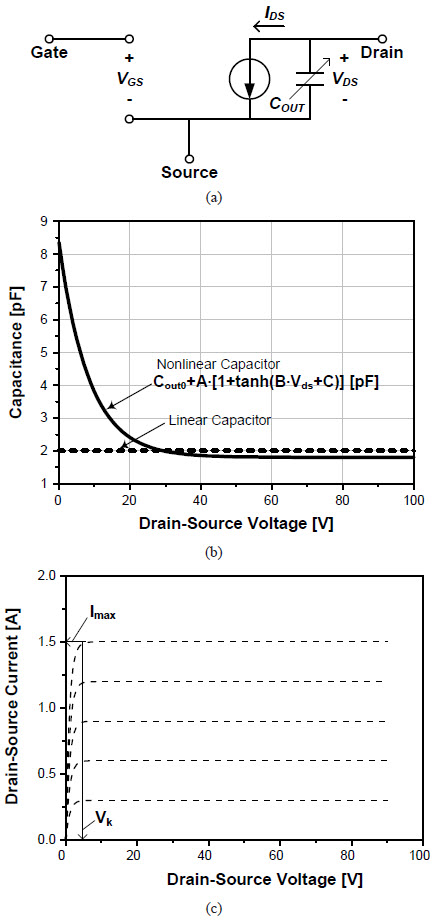Fig. 1. (a) Simpliﬁed transistor equivalent circuit model. (b) Capacitances for the linear and nonlinear Couts. (c) DC-IV characteristic.

#### II. ANALYSIS FOR OPERATION CHARACTERISTICS OF A CLASS-J AMPLIFIER

A. Ideal Transistor Model for Simulations

To analyze the fundamental behaviors of the PAs, we have constructed a simpliﬁed transistor equivalent circuit model in the Agilent Advanced Design System (ADS) using the sym-bolically deﬁned device, as shown in Fig. 1(a). To simplify the analysis, the transistor model contains two essential nonlinear parts: Cout and the drain current source. Cout represents all nonlinear capacitors of the transistor output, including the drain-source capacitor Cds and gate-drain capacitor Cgd. Even though Cds and Cgd are modulated according to both the drain-source and the gate-source voltages, we assume only a dependence on the drain-source voltage in this model. Thus, Cout is given by

Cout (Vds) = Cout0 +A·[1 + tanh (B · Vds + C)] [pF] , (1)

where Cout0, A, B, and C are summarized in Table I. Fig. 1(b) illustrates the characteristic of the nonlinear Cout ac-cording to the drain-source voltage. The capacitance increases dramatically as the drain-source voltage becomes small. The drain-source current is given by

where gm is the trans-conductance, and Vgs,max is the gate-source voltage when Ids is equal to Imax. For simplicity, we assume the pinch-off voltage is zero. As depicted in Fig. 1(c), the transistor model exhibits strongly nonlinear effects of cutoff and saturation. In the intermediate region between the cutoff and saturation, the transistor provides a highly linear operation. In particular, gm and Imax are set to 1 S and 1.5 A, respectively, in this model, and Vk is about 4 V. These parameters are based on the model of the Cree GaN HEMT CGH60015 used for the implementation. It is well known that the input nonlinear capacitor Cgs has nonlinear characteristic, which results in the generation of harmonic components –, , . However, compared with the output nonlinear capacitor, Cgs has a minor effect on the performance, as will be described in Section III, so the effect of Cgs nonlinearity is eliminated in this model.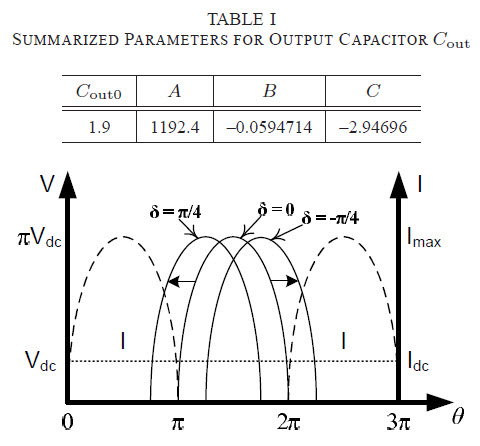Fig. 2. Half-sinusoidal voltage and current waveforms for the various phase differences (In particular, δ = π/4 represents the Class-J PA).

B. Class-J Ampliﬁer with Linear Output Capacitor

First, we review the operation of Class-J ampliﬁer with linear Cout. Linear Cout refers to a constant capacitance, as shown in Fig. 1(b). Class-J ampliﬁer can be characterized by half-sinusoidal voltage and current waveforms with a phase shift between them, as depicted in Fig. 2. These waveforms can be expressed by

where δ is a phase difference between the voltage and current from the normal 180◦. Thus, the load impedances of each harmonic frequencies can be calculated by

where n denotes the nth frequency component. For Imax = 1 and Vdc = 1/π, the fundamental and second-harmonic loads are given by

For Class-J ampliﬁer, δ is π/4 because the second harmonic loading is made by the capacitive component. Thus, to shape the half-sinusoidal voltage waveform, the fundamental load impedance is set to 1∠(π/4). This leads to the degradation of the output power by a factor of cos (π/4).

The operation of the Class-J PA is further investigated by using the model described in Section II-A. Since the Class-J operation biased at the Class-B condition is assumed in this work, the Class-B ampliﬁer is also simulated for comparison purpose. For the Class-B design, the optimum fundamental load impedance is chosen to obtain the maximum output power.

In the simulation, Vdc is set to 30 V, so Ropt is chosen to be 34.6 Ω.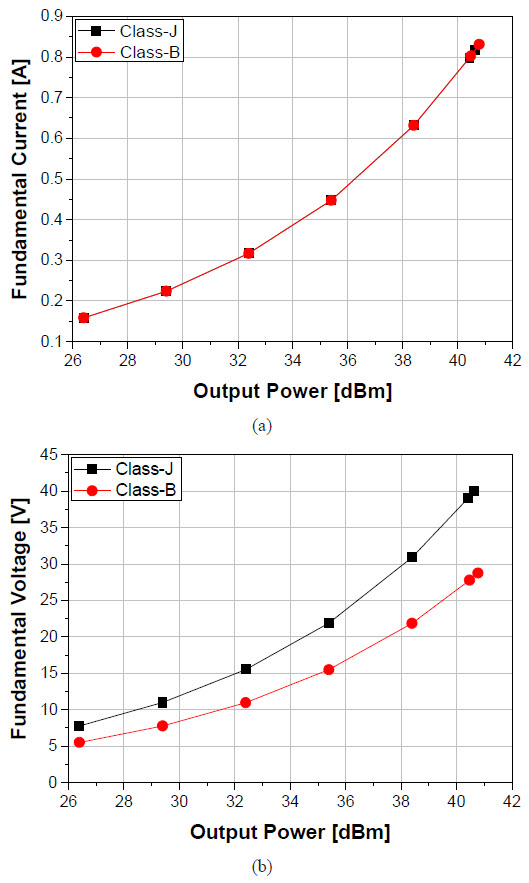Fig. 4. Fundamental (a) current and (b) voltage components for the Class-B and Class-J ampliﬁers with the linear Cout.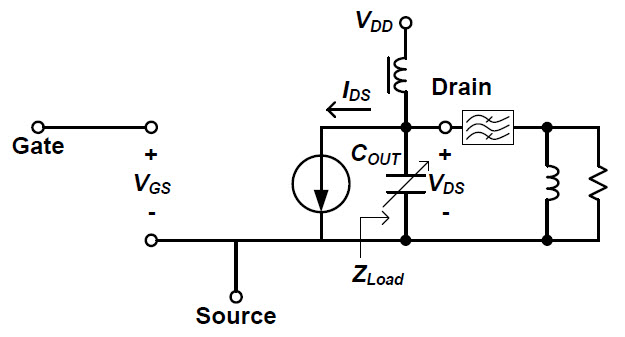Fig. 6. Simulation setup to investigate the behavior of the ampliﬁers and the harmonic-generation property of the nonlinear Cout.

For the Class-J ampliﬁer, the voltage waveform is half-sinusoidal. It consists of all even harmonic voltage compo-nents. However, the voltage components at the higher-order frequencies are assumed to be zero because of the device’s large output capacitor. We believe that, in the real design environment, the half-sinusoidal voltage waveform is mainly made up the DC, fundamental, and second-harmonic volt-age components. Among the various half-sinusoidal voltage waveforms manipulated by up to second-harmonic component, the “maximum-voltage gain” condition is assumed in this study – . Accordingly, the magnitude of the funda-mental load impedance should be set to √2Ropt because the maximum value of the fundamental voltage extracted from the half-sinusoidal waveform is increased by a factor of √2 above that of Class-B ampliﬁer. In addition, to properly shape the half-sinusoidal voltage waveform, the appropriate amount of the second-harmonic voltage is required. In particular, the “maximum-voltage gain” condition can be achieved when the ratio of the second-harmonic to the fundamental voltage is −√2/4. Thus, assuming the ideal half-sinusoidal current having the fundamental current of max 2 and the second-harmonic current of 2Imax/3π, the required second-harmonic load impedance is given by

Consequently, the load condition for Class-J ampliﬁer is represented by

In the simulation of the Class-J ampliﬁer, the fundamental and second-harmonic load impedances are selected to be 34.6 +
j34.6 Ω and −j40.768 Ω, respectively.

C. Class-J Ampliﬁer with Nonlinear Output Capacitor

Fig. 3 depicts the simulated time-domain voltage and current waveforms and load-lines for the designed Class-J and Class-B ampliﬁers with linear Cout. Because of the complex fundamental load impedance, the Class-J ampliﬁer provides a loadline having “looping-characteristic”. Fig. 4 shows the fundamental current and voltage components for the Class-B and Class-J ampliﬁers. In the saturated region, the fundamental current of the Class-B is slightly larger than that of the Class-J, because the Class-J has an asymmetric current waveform due to the complex fundamental load impedance, as shown in Fig. 3(a). However, the bifurcated current of the Class-B requires slightly larger DC current than the Class-J, and the efﬁciency of the Class-B at the highly saturated region is slightly lower than that of the Class-J ampliﬁer, as depicted in Fig. 5. Although the fundamental voltage of the Class-J ampliﬁer is increased by a factor of √2 compared to the Class-B, as shown in Fig. 4(b), the output power and efﬁciency of the Class-J are equal to those of the Class-B ampliﬁer due to the phase mismatch between the current and voltage waveforms by 45◦. In short, although the Class-B and Class-J ampliﬁers with linear Cout have different fundamental loads and harmonic terminations, the performances of the two PAs are nearly the same except at the highly saturated condition. However, even at the highly saturated condition, the difference is very small.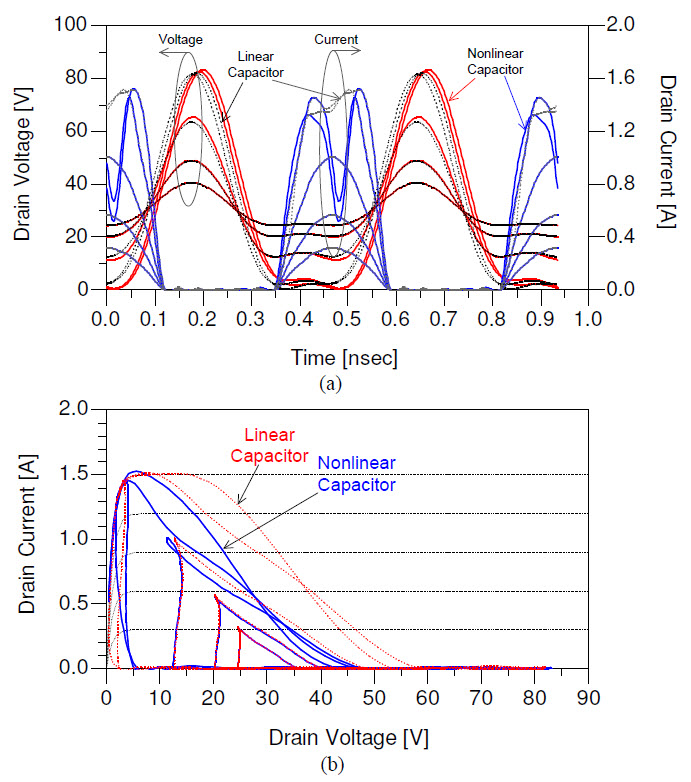Fig. 7. Simulated (a) time-domain voltage and current waveforms and (b) load-lines for the Class-J ampliﬁers with the linear and nonlinear Couts.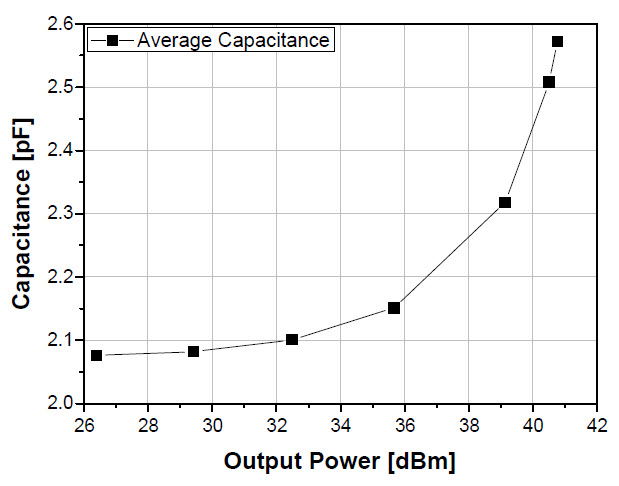Fig. 8. Average capacitance for the Class-J with the nonlinear Cout according to the power level.

Although Section II-B explains clearly the fundamental operation of the Class-J ampliﬁer and provides the proper load conditions, it does not describe the real behavior of the ampliﬁer because Cout is not a linear element but a nonlinear element, as shown in Fig. 1(b). In this section, the basic operation of a Class-J ampliﬁer with the nonlinear Cout is investigated using the setup shown in Fig. 6. Here, we deﬁne the load impedance at the current source ZLoad and the capacitance is tuned for the fundamental frequency at low power level. Fig. 7 represents the simulated time-domain voltage and current waveforms and load-lines of the Class-J ampliﬁers with linear and nonlinear Couts, and Fig. 8 describes the average capacitance according to the output power level for the Class-J ampliﬁer with the nonlinear Cout. In the low-power region, below 32 dBm (1.6 W) of the output power, the swing of the drain voltage is within the range where the nonlinear Cout could be regarded as a linear capacitance, as shown in Fig. 7 and Fig. 1. At the output power of 32 dBm (1.6 W), the voltage is varied from 20 V to 50 V so that the average capacitance remains around 2.1 pF. Therefore, the waveforms and load-lines with the nonlinear Cout case are the same as those with the linear Cout case. This results in nearly the same performances for the output power and efﬁciency, as shown in Fig. 9. In addition, the output fundamental and second-harmonic load impedances for both the linear and nonlinear Couts are the same, properly shaping the half-sinusoidal voltage waveforms, as described in Fig. 10. However, as the output power increases above 32 dBm (1.6 W), the average capacitance also enlarges with a large capacitance at a low voltage. Thus, as shown in Fig. 7(a), the current waveform has a large dip at the middle, generating a large third-harmonic current and enhancing the efﬁciency. Moreover, the magnitude of the fundamental load impedance increases, but the phase decreases as depicted in Fig. 10. As a result, unlike the Class-J ampliﬁer with the linear Cout, the voltage waveform moves toward the direction to reduce the phase difference between the current and voltage waveforms while maintaining the half-sinusoidal shape of the voltage. This results in enhanced output power and efﬁciency performances compared to the Class-J ampliﬁer with the linear Cout. What is interesting here is that these waveforms can be made with δ of less than 45◦. However, from (5), δ less than 45◦ leads to a negative resistance value of the second harmonic impedance. This means that the nonlinear Cout generates the second-harmonic voltage component. This phenomenon cannot be expected from the operation of a Class-J ampliﬁer with a linear Cout.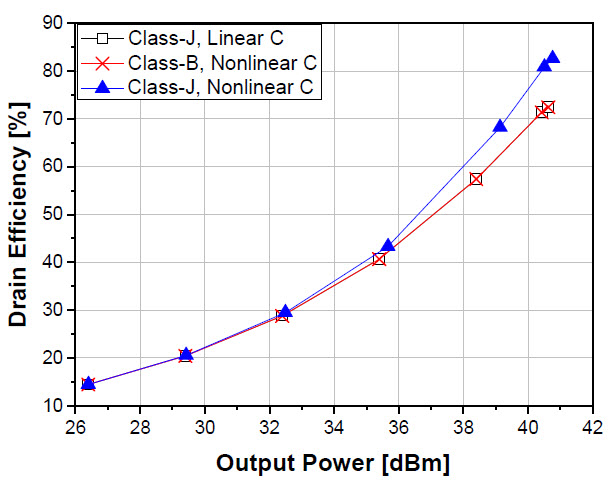Fig. 9. Simulated efﬁciencies for the Class-J ampliﬁer with a linear Cout and the Class-B and -J ampliﬁers with the nonlinear Cout according to the power level.

Fig. 11 shows the simulation results to demonstrate the harmonic generation of the nonlinear Cout. The simulation is carried out using the circuit in Fig. 6. The simulation results include the current ﬂowing through the linear and nonlinear Cout and the resultant capacitor voltage waveforms in the time-domain and frequency-domain. Differently from the linear capacitor, the nonlinear capacitor generates a voltage waveform consisting of the fundamental and harmonic voltage components. Although only the fundamental current is injected to the capacitor, the voltage contains a large second-harmonic component with small higher-order frequency components. Since the transistor can be regarded as a voltage controlled current source, the current ﬂowing through the capacitor is determined by the input voltage. The voltage across the capacitor is proportional to the integral of the current, the charge in the capacitor, scaled by the capacitance. It can be expressed by

Q (tx) indicates the charge on Cout until tx. As Q (tx) decreases with negative i (t), Cout increases rapidly. Around the bottom of the voltage, the output voltage VDS (tx) cannot change much because of the large variation of Cout with the limited current drive. That is, Q (tx) /Cout (VDS (tx)) remains nearly constant, VDD, around the region. As a result, the voltage waveform has a ﬂattened characteristic in the low voltage region, like the half-sinusoidal shape, as shown in Fig. 11(b). Thus, the second-harmonic impedance can be in the negative resistance region as shown in Fig. 10 because the second-harmonic component in the voltage waveform is generated not by the second-harmonic current and load but by the nonlinear Cout. This second harmonic component allows the phase difference between the fundamental current and voltage less than 45◦. Additionally, the harmonic components generated by the nonlinear capacitor can be varied according to the nonlinear Cout proﬁle. The more the nonlinear capacitor changes, the more the second-harmonic is generated. If the second-harmonic load impedance attached parallel to the non-linear Cout is larger than the impedance of the nonlinear Cout, the half-sinusoidal voltage waveform can be maintained. The second-harmonic current component generated by the current source can assist this behavior, generating the half-sinusoidal voltage waveform. However, the saturated current with a large dip at the middle has a signiﬁcant third-harmonic and rather small second-harmonic current. Together with the low harmonic impedance, the second-harmonic voltage is mainly built up by the harmonic voltage generation of the nonlinear capacitor. We will revisit this issue in the simulation using a real device model in Section III.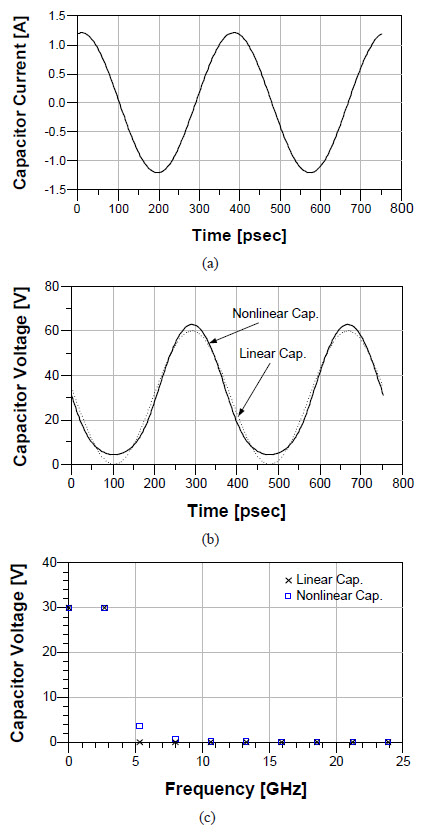Fig. 11. Simulation results to demonstrate the harmonic generation of the nonlinear Cout. (a) Current ﬂowing from the linear and nonlinear Couts. The resultant capacitor voltage waveforms in the (b) time- and (c) frequency-domain.

D. Optimization of Class-J Ampliﬁer with Nonlinear Output Capacitor: Saturated Ampliﬁer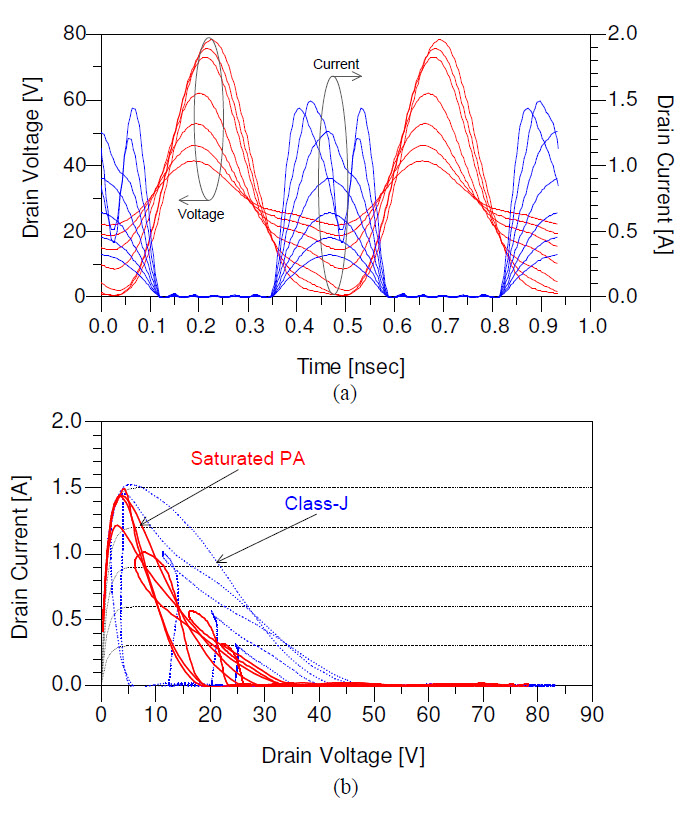Fig. 12. Simulated (a) time-domain voltage and current waveforms for the saturated ampliﬁer and (b) load-lines for the Class-J and saturated ampliﬁers.

Fig. 12 shows the simulated time-domain voltage and cur-rent waveforms and load-lines for the Class-J and saturated ampliﬁers with nonlinear Cout. Unlike the waveforms of the Class-J ampliﬁer depicted in Fig. 7(a), the phase difference between the current and voltage is nearly zero due to the resistive fundamental load impedance, as shown in Fig. 13. Moreover, since the second-harmonic voltage to shape the half-sinusoidal voltage waveform is generated mainly by the nonlinear Cout, the second-harmonic impedance remains in the negative resistance region. However, in the low-power region where the nonlinear Cout can be regarded as a linear capacitor, the phase of the fundamental-load impedance of the saturated ampliﬁer is not 45◦ while the phase of the second-harmonic is −90◦, so it does not provide the half-sinusoidal voltage waveform. Fig. 14 shows the simulated efﬁciencies for the Class-J and saturated ampliﬁers. Since the saturated ampliﬁer has a smaller phase mismatch between the voltage and current compared to the Class-J ampliﬁer, it produces a higher output power and efﬁciency than the Class-J ampliﬁer.

In short, for the linear Cout, the Class-J ampliﬁer does not have any merit compared to the Class-B ampliﬁer with respect to the output power and efﬁciency because of the phase mismatch between the voltage and current waveforms. However, for the nonlinear Cout case, the Class-J ampliﬁer has a chance to improve the performance because the nonlinear capacitor generates harmonic-voltage components, especially the second-harmonic voltage component. Thus, the phase difference can be reduced below −45◦. A further improvement can be achieved by selecting a purely resistive load for the fundamental frequency to eliminate the phase mismatch between the voltage and current, while maintaining the half-sinusoidal voltage waveform. This can be carried out if the external second-harmonic impedance is greater than the level of the nonlinear Cout, which is a saturated PA, the further optimized version of the Class-J ampliﬁer for highly efﬁcient operation.

#### III. IMPLEMENTATION AND EXPERIMENTAL RESULTS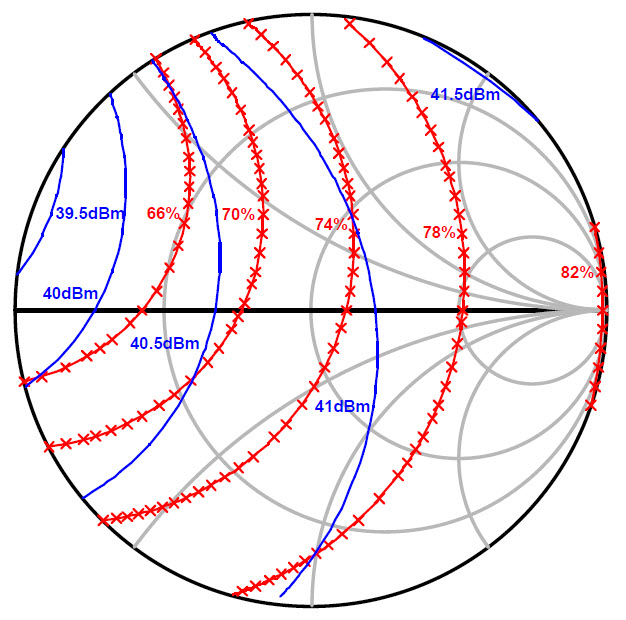Fig. 15. Simulated second-harmonic load-pull contours of the output power and efﬁciency when the fundamental and third-harmonic impedance are set to 18.23 + j25.15 Ω and 0 Ω, respectively, at the drain pad of the bare chip.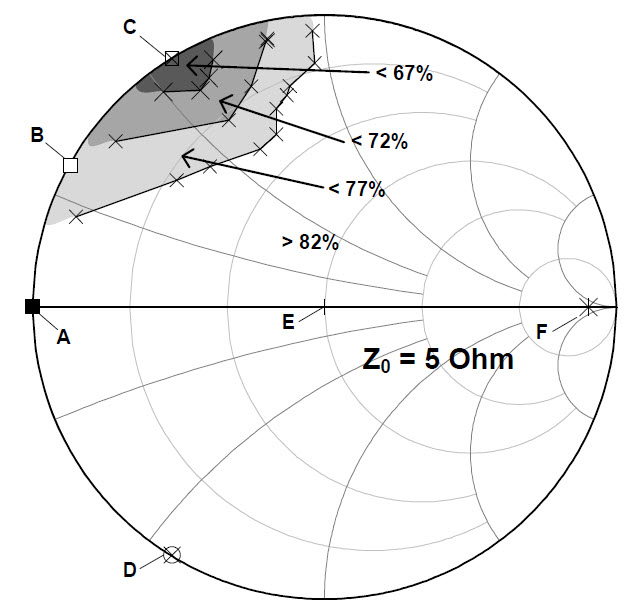Fig. 16. Simulated second-harmonic source-pull contours of the efﬁciency when the fundamental source impedance is conjugate-match condition and the output load terminations are set to the condition of the saturated PA. The characteristic impedance of the smith chart is 5 Ω.

To validate the voltage waveform shaping by the nonlinear Cout and the highly efﬁcient operation, a saturated ampli-ﬁer was designed and implemented at 2.14 GHz using a Cree GaN HEMT CGH40010 package device containing a CGH60015 bare chip. Since the commercial device model includes packaging effects, due to bonding wires, package leads, and parasitics, the simulation is conducted using a bare-chip model to show the inherent operation of the saturated PA. In addition, the simulation for the implementation is conducted using the model of the packaged-device. Fig. 15 shows the simulated second-harmonic load-pull contours of the output power and efﬁciency when the fundamental and third-harmonic impedances are set to 18.23 + j25.15 Ω and 0 Ω, respectively, at the drain pad of the bare chip. The load-pull simulation was carried out using Agilent ADS 2008 Update 1. The device model, CGH60015 and package information, were provided by Cree Inc.. As mentioned in Section II, a high efﬁciency and output power can be achieved when the second-harmonic load impedance is large, proving that the nonlinear Cout is enough to shape the voltage to the half-sinusoidal. Since a large second-harmonic load impedance provides a high efﬁciency, it is set to 2 + j80 Ω. For the high efﬁciency and output power operation, a fundamental-load impedance at the dependent current source is determined to be about 60 Ω. Since the efﬁciency changes a little according to the third-harmonic matching impedance, the impedance is set to 0.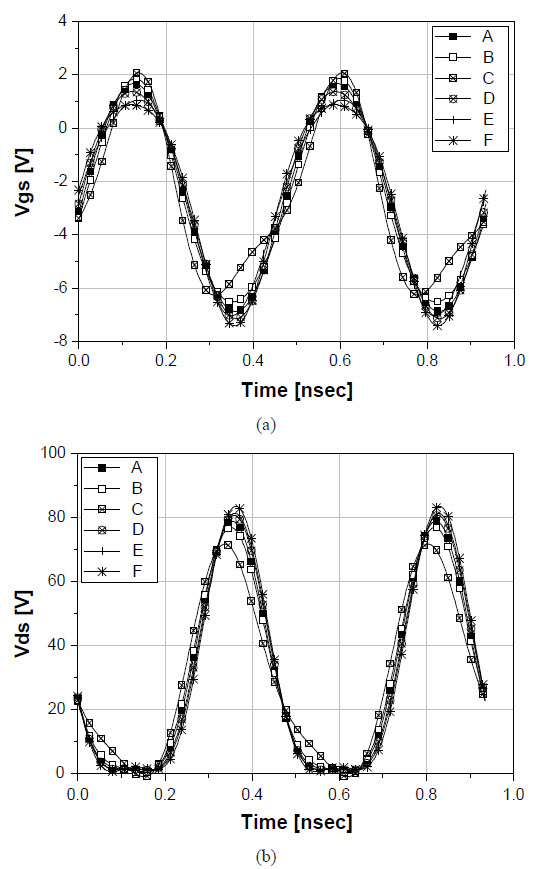Fig. 17. Simulated (a) Vgs and (b) Vds waveforms at the marked points on the Smith chart in Fig. 16 (A = 0 Ω, B = 0.01 + j1.3 Ω, C =0.01 + j2.8 Ω, D = 0.01 − j2.8 Ω, E = 5 Ω, and F = 100 Ω).

So far, the fundamental and harmonic-load impedances at the output have been considered to achieve high power and efﬁciency capabilities, and the fundamental input component is conjugately matched. However, it has been observed that the input harmonic terminations should be properly placed due to the nonlinear input capacitor Cgs. In particular, to preserve the sinusoidal input drive at the gate, the input harmonic terminations are frequently set to be short-circuit , . On the other hand, in – , they make an effort to shape the output voltage waveform by using the nonlinear effect of Cgs. To ﬁnd the optimum input termination for the second harmonic, the source-pull simulation for the second harmonic is carried out, as shown in Fig. 16. During the simulation, the fundamental source impedance is conjugately matched, and the output load terminations are set to the condition of the saturated ampliﬁer. A simulation of source-pull for the second-harmonic termination indicates only a minor effect on the efﬁciency if the termination is not in the gray colored region. This result supports that the output voltage waveform is mainly shaped by the nonlinear output capacitor. Fig. 17 shows the simulated Vgs and Vds waveforms for second harmonic impedances at the marked points on the Smith chart in Fig. 16. Except for the points marked by A and C, the Vgs waveforms are very similar due to the harmonic voltage generation of Cgs. Not just for these points, almost regions of the smith chart, except for the gray colored part, provide the analogous Vgs waveforms. For the point marked by A, due to the short-circuit for the second harmonic, the sinusoidal voltage is presented. In spite of the different Vgs waveforms, the output voltages at the drain are similar shapes. Those waveforms clearly show that the output voltage shape is not much affected by the harmonic generation of Cgs while considering the nonlinear Cds. However, even though most of regions provide the same performance, the output power and efﬁciency deteriorated when the source impedance for the second harmonic is located at the gray-colored region. In this region, the conduction angle of the drain current is reduced, resulting in low output power and efﬁciency. Therefore, in this work, the input termination for the second harmonic is set to the short-circuit.Fig. 18. (a) Simulated time-domain voltage waveforms of the saturated am-pliﬁer and Class-B PA. (b) Fundamental and second-harmonic load impedance trajectories of the saturated ampliﬁer and Class-B PA according to the power levels.

Fig. 18 indicates the simulated time-domain voltage waveform and the fundamental and second-harmonic load impedance trajectories according to the power level. Like the simulation conducted in Section II-D, the half-sinusoidal voltage waveform is achieved at the high power level where a large amount of the second harmonic is generated by the nonlinear Cout, so that the second-harmonic impedance remains in the negative resistance region. For comparison, the Class-B ampliﬁer is also simulated using the same device. To provide the sinusoidal voltage waveform, all harmonics are short-circuited. In addition, the fundamental-load impedance of the Class-B PA is set to 36.5 Ω at the maximum power level to provide the full swings of the voltage and current waveforms. Fig. 19 shows the simulated gain and efﬁciency comparisons between the saturated PA and Class-B ampliﬁer. Because the load impedance of the saturated PA is larger than that of the Class-B ampliﬁer, the saturated PA provides higher gain than the Class-B’s one in the low power region. At the high power level, compared with the Class-B ampliﬁer, the saturated PA delivers the better efﬁciency performance with comparable output power, resulting from the second-harmonic manipulation caused by the nonlinear Cout. In particular, the maximum efﬁciency of the saturated PA is 81.5%, which is an improvement of 9.3%.

Fig. 20(a) shows a photograph of the designed PA imple-mented on a Taconic TLY-5 substrate with εr = 2.2 and thickness of 31 mil. The detailed microstrip dimensions are shown in Fig. 20(b). In the experiment, the gate bias is set to –3.1 V (IdsQ = 94 mA) at a supplied drain voltage of 30 V. Unlike the conventional high efﬁciency PAs, any special harmonic-loading circuit is not used in the output matching. The simulated and measured output power, efﬁciency, and gain characteristics for a CW signal are well matched, as shown in Fig. 21. In particular, the implemented PA provides a maximum PAE of 77.3% at a saturated output power of 40.6 dBm (11.5 W). Fig. 22 depicts the measured adjacent channel leakage ratio (ACLR) and PAE for LTE signal with 6.6 dB PAPR and 10 MHz signal bandwidth. The proposed PA delivers a PAE of 42.3% and a power gain of 21 dB at an average output power of 34.2 dBm (2.6 W). To validate potential of the proposed PA as a main block of a linear power ampliﬁer (LPA), the linearization of the PA is carried out by employing the digital feedback predistortion technique (DPBPD) . Fig. 23 shows the measured output spectra before and after the linearization. The ACLR at an offset of 7.5 MHz is –45.1 dBc, which is an improvement of 23 dB at an average output power of 34.2 dBm (2.6 W). The linearization results are summarized in Table II. These experimental results clearly show that the proposed saturated ampliﬁer can provide excellent efﬁciency without any special harmonic loading circuit. Moreover, by applying the lineariza-tion technique, it is well suited to be a highly efﬁcient main ampliﬁer of a LPA for use in a LTE transmitter.

Comparison of the performance among the designed PA with state-of-the-art results for high-efﬁciency is summarized in Table III. The comparison shows the excellent performance of the saturated PA, the optimized Class-J PA, without any harmonic control circuitry.

#### IV. CONCLUSION

The operation principles of Class-J ampliﬁers with linear and nonlinear Couts are analyzed using a simple transistor model in an ADS simulator. The performance of the Class-J PA with linear Cout is comparable to that of the conventional Class-B ampliﬁer. However, due to the harmonic generation property of the nonlinear Cout, the half-sinusoidal voltage waveform can be properly shaped while the phase overlap be-tween the voltage and current components are reduced below 45◦. This allows the improvement of the efﬁciency beyond that of a Class-J ampliﬁer with a linear Cout. The further optimization of the ampliﬁer can be carried out by adopting the phase difference to zero degree using the purely resis-tive fundamental load impedance. It minimizes the dissipated power caused by the concurrent non-zero voltage and current while maintaining the half-sinusoidal voltage waveform. Since the voltage shaping is achieved by the nonlinear Cout, efforts to control the harmonic components are unnecessary. If the external harmonic load impedances are larger than the level of the capacitor, a highly efﬁcient voltage waveform is obtained. This is supported by the ADS simulation using both ideal and real models of the transistor. This is the optimized version of Class-J PA, which is the saturated PA we have reported. A highly efﬁcient saturated PA is implemented by using a Cree GaN HEMT CGH40010 device at 2.14 GHz. It provides a PAE of 77.3% and a saturated output power of 40.6 dBm (11.5 W) without any special harmonic loading network.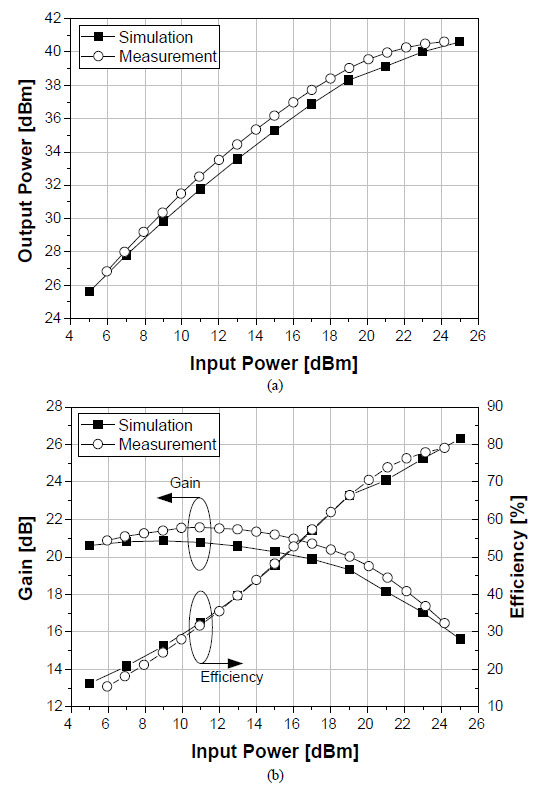Fig. 21. Simulated and measured (a) output power, (b) efﬁciency and gain characteristics for a CW signal.

ACKNOWLEDGEMENT

The authors would like to thank Cree Inc. for providing the GaN HEMT transistors and models used in this work.

REFERENCES

 F. H. Raab, P. Asbeck, S. Cripps, P. B. Kenington, Z. B. Popovi´c, N. Pothecary, J. F. Sevic, and N. O. Sokal “Power ampliﬁers and transmitters for RF and microwave,” IEEE Trans. Microw. Theory Tech., vol. 50, no. 3, pp. 814–826, Mar. 2002.

 J. Choi, D. Kang, D. Kim, J. Park, B. Jin, and B. Kim, “Power ampliﬁers and transimitters for next generation mobile handset,” J. Semicond. Technol. Sci., vol. 9, no. 4, pp. 249–256, Dec. 2009.

 S. C. Cripps, RF Power Ampliﬁers for Wireless Communications, 2nd Edition, Norwood, MA: Artech House, 2006.

 F. H. Raab, “Class-F power ampliﬁers with maximally ﬂat waveforms,” IEEE Trans. Microw. Theory Tech., vol. 45, no. 11, pp. 2007–2012, Nov. 1997.

 S. Gao, “High-efﬁciency class-F RF/microwave power ampliﬁers,” IEEE Microw. Mag., vol. 7, no. 1, pp. 40–48, Feb. 2006.

 Y. Y. Woo, Y. Yang, and B. Kim, “Analysis and experiments for high-efﬁciency class-F and inverse class-F power ampliﬁers,” IEEE Trans. Microw. Theory Tech., vol. 54, no. 5, pp. 1969–1974, May 2006.

 N. O. Sokal and A. D. Sokal, “Class-E: A new class of high-efﬁciency tuned single-ended switching power ampliﬁers,” IEEE J. Solid-State Circuits, vol. SC-10, pp. 168–176, Jun. 1975.

 F. H. Raab, “Idealized operation of the class-E tuned power ampliﬁer,” IEEE Trans. Circuits Syst., vol. 24, no. 12, pp. 725–735, Dec. 1977.

 S. C. Cripps, P. J. Tasker, A. L. Clarke, J. Lees, and J. Benedikt, “On the continuity of high efﬁciency modes in linear RF power ampliﬁers,” IEEE Microw. Wireless Compon. Lett., vol. 19, no. 10, pp. 665–667, Oct. 2009.

 P. Colantonio, F. Giannini, G. Leuzzi, and E. Limiti, “High efﬁciency low-voltage power ampliﬁer design by second-harmonic manipulation,” Int. J. RF Microw. Comput.-Aided Eng., vol. 10, no. 1, pp. 19–32, 2000.

 ——, “Class G approach for high efﬁciency PA design,” Int. J. RF Microw. Comput.-Aided Eng., vol. 10, no. 6, pp. 366–378, 2000.

 ——, “Multiharmonic manipulation for highly efﬁcient microwave power ampliﬁers,” Int. J. RF Microw. Comput.-Aided Eng., vol. 11, no. 6, pp. 366–384, 2001.

 P. Wright, J. Lees, J. Benedikt, P. J. Tasker, and S. C. Cripps, “A methodology for realizing high efﬁciency Class-J in a linear and broadband PA,” IEEE Trans. Microw. Theory Tech., vol. 57, no. 12, pp. 3196–3204, Dec. 2009.

 ——, “An efﬁcient, linear, broadband Class-J mode PA realised using RF wavefrom engineering,” in IEEE MTT-S Int. Microw. Symp. Dig., Jun. 2009, pp. 653–656.

 H. Nemati, C. Fager, M. Thorsell, and H. Zirath, “High-efﬁciency LDMOS power-ampliﬁer design at 1 GHz using an optimized transistor model,” IEEE Trans. Microw. Theory Tech., vol. 57, no. 7, pp. 1647–1954, Jul. 2009.

 Y. Yang, Y. Y. Woo, J. Yi, and B. Kim, “A new empirical large-signal model of Si LDMOSFETs for high-power ampliﬁer design,” IEEE Trans. Microw. Theory Tech., vol. 49, no. 9, pp. 1626–1633, Sep. 2001.

 R. Pengelly, B. Million, D. Farrell, B. Pribble, and S. Wood, “ Application of non-linear models in a range of challenging GaN HEMT power ampliﬁer design,” in IEEE MTT-S Int. Microw. Symp. Dig., Jun. 2008, [CD ROM].

 M. J. Chudobiak, “The use of parasitic nonlinear capacitors in class-E ampliﬁers,” IEEE Trans. Circuits Syst. I, vol. 41, no. 12, pp. 941–944, Dec. 1994.

 T. Suetsugu, and M. K. Kazimierczuk, “Comparison of class-E ampliﬁer with nonlinear and linear shunt capacitance,” IEEE Trans. Circuits Syst. I, vol. 50, no. 8, pp. 1089–1097, Aug. 2003.

 A. Mediano, P. Molina, and J. Navarro, “ Class E RF/microwave power ampliﬁer: linear “equivalent” of transistor’s nonlinear output capacitance, normalized design and maximum operating frequency vs. output capacitance,” in IEEE MTT-S Int. Microw. Symp. Dig., Jun. 2000, pp. 783–786.

 A. Mediano, P. M. Gaud´o, and C. Bernal, “Design of class E ampliﬁer with nonlinear and linear shunt capacitances for any duty cycle,” IEEE Trans. Microw. Theory Tech., vol. 55, no. 3, pp. 484–492, Mar. 2007.

 M. K. Kazimierczuk and W. A. Tabisz, “Class C-E high-efﬁciency tuned power ampliﬁer,” IEEE Trans. Circuits syst., vol. 36, no. 3, pp. 421–428, Mar. 1989.

 H. L. Krauss, C. W. Bostian, and F. H. Raab, Solid State Radio Engineering, New York: Wiley, 1980.

 B. Kim, J. Moon, and J. Kim, “Highly efﬁcient saturated power ampliﬁer assisted by nonlinear output capacitor,” in IEEE MTT-S Int. Microw. Symp. Dig. Workshop, May 2010.

 J. Kim, J. Kim, J. Moon, J. Son, I. Kim, S. Jee, and B. Kim, “Saturated power ampliﬁer optimized for efﬁciency using self-generated harmonic current and voltage,” IEEE Trans. Microw. Theory Tech., submitted for publication.

 P. M. White, “Effect of input harmonic terminations on high efﬁciency class-B and class-F operation of PHEMT devices,” in IEEE MTT-S Int. Microw. Symp. Dig., Jun. 1998, pp. 1611–1614.

 S. Watanabe, S. Takatuka, and K. Takagi, “Simulation and experimental results of source harmonic tuning on linearity of power GaAs FET under class AB operation,” in IEEE MTT-S Int. Microw. Symp. Dig., Jun. 1996, pp. 1771–1774.

 Y. Y. Woo, J. Kim, J. Yi, S. Hong, I. Kim, J. Moon, and B. Kim, “Adaptive digital feedback predistortion technique for linearizing power ampliﬁer,” IEEE Trans. Microw. Theory Tech., vol. 55, no. 5, pp. 932–940, May 2007.

 N. Ui and S. Sano, “A 45% drain efﬁciency, –50 dBc ACLR GaN HEMT class-E ampliﬁer with DPD for W-CDMA base station,” in IEEE MTT-S Int. Microw. Symp. Dig., Jun. 2006, pp. 718–721.

 H. G. Bae, R. Negra, S. Boumaiza, and F. M. Ghannouchi, “High-efﬁciency GaN class-E power ampliﬁer with compact harmonic-suppression network,” in Proc. 37th IEEE Eur. Microw. Conf., Oct. 2007, pp. 1093–1096.

 D. Schmelzer and S. I. Long, “A GaN HEMT class F ampliﬁer at 2 GHz with > 80% PAE,” IEEE J. Solid-State Circuits, vol. 42, no. 10, pp. 2130–2136, Oct. 2007.

 P. Wright, A. Scheikh, C. Roff, P. J. Tasker, and J. Benedikt, “Highly efﬁcient operation modes in GaN power transistors delivering upwards of 81% efﬁciency and 12 W output power,” in IEEE MTT-S Int. Microw. Symp. Dig., Jun. 2008, pp. 1147–1150.

 S. Bensmida, O. Hammi, and F. M. Ghannouchi, “High efﬁciency digitally linearized GaN based power ampliﬁer for 3G applications,” in Proc. 2008 IEEE Radio and Wireless Symp., Jan. 2008, pp. 419–422.

 M. P. van der Heijden, M. Acar, and J. Vromans, “A compact 12-watt high-efﬁciency 2.1–2.7 GHz class-E GaN HEMT power ampliﬁer for base stations,” in IEEE MTT-S Int. Microw. Symp. Dig., Jun. 2009, pp. 657–660.

Junghwan Moon (S’07) received the B.S. degree in electrical and computer engineering from the University of Seoul, Seoul, Korea, in 2006 and is currently working toward the Ph.D. degree at the Pohang University of Science and Technology (POSTECH), Pohang, Gyungbuk, Korea.

His current research interests include highly linear and efﬁcient RF PA design, memory-effect com-pensation techniques, digital predistortion (DPD) techniques, and wideband RF PA design.

Mr. Moon was the recipient of the Highest Efﬁ-ciency Award at Student High-Efﬁciency Power Ampliﬁer Design Competition in IEEE MTT-S International Microwave Symposium (IMS), 2008.

Jungjoon Kim received the B.S. degree in electrical engineering from Han-Yang University, Ansan, Korea, in 2007, and the Master degree in electrical engineering from the Pohang University of Science and Technology (POSTECH), Pohang, Gyungbuk, Korea, in 2009. He is currently working toward the Ph.D. degree at the POSTECH, Pohang, Gyungbuk, Korea.

His current research interests include RF PA de-sign and supply modulator design for highly efﬁcient transmitter system.

Bumman Kim (M’78-SM’97-F’07) received the Ph.D. degree in electrical engineering from Carnegie Mellon University, Pittsburgh, PA, in 1979.

From 1978 to 1981, he was engaged in ﬁber-optic network component research with GTE Laboratories Inc. In 1981, he joined the Central Research Labo-ratories, Texas Instruments Incorporated, where he was involved in development of GaAs power ﬁeld-effect transistors (FETs) and monolithic microwave integrated circuits (MMICs). He has developed a large-signal model of a power FET, dual-gate FETs for gain control, high-power distributed ampliﬁers, and various millimeter-wave MMICs. In 1989, he joined the Pohang University of Science and Technology (POSTECH), Pohang, Gyungbuk, Korea, where he is a POSTECH Fellow and a Namko Professor with the Department of Electrical Engineering, and Director of the Microwave Application Research Center, where he is involved in device and circuit technology for RF integrated circuits (RFICs). He has authored over 300 technical papers.

Prof. Kim is a member of the Korean Academy of Science and Tech-nology and the National Academy of Engineering of Korea. He was an associate editor for the IEEE TRANSACTIONS ON MICROWAVE THEORY AND TECHNIQUES, a Distinguished Lecturer of the IEEE Microwave Theory and Techniques Society (IEEE MTT-S), and an AdCom member.

This material is posted here with permission of the IEEE. Such permission of the IEEE does not in any way imply IEEE endorsement of any of Cree’s products or services. Internal or personal use of this material is permitted. However, permission to reprint/republish this material for advertising or promotional purposes or for creating new collective works for resale or redistribution must be obtained from the IEEE by writing to pubs-permissions@ieee.org By choosing to view this document, you agree to all provisions of the copyright laws protecting it.

Stay Informed
Sales & Technical Support
Request Samples
Visit the Sample Center
Large Signal Models
Stay Informed
Sales & Technical Support
Large Signal Models

## More Articles

View All
RF
|
Aerospace & Defense

### Thermal Considerations for High-Power GaN RF Amplifiers

Technical Articles

### Improving Pulse Fidelity in RF Power Amplifiers

A radar system designer’s most coveted objectives are achieving a long range, adequate resolution to distinguish objects in close proximity to each other, and the ability to not only determine target velocities but target types in order to help differentiate friendlies from adversaries.A combination of both approaches is essential, and engineers can design for peak power points of the load-pull simulation while also paying attention to other parts of the circuit for baseband signal fidelity.